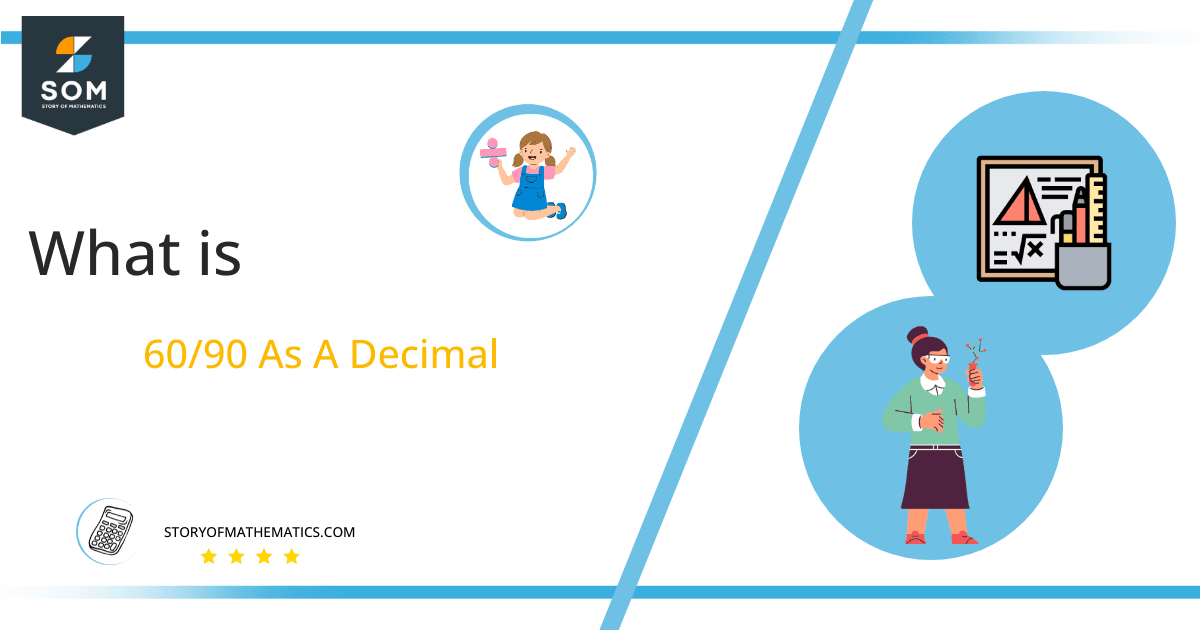# What Is 60/90 as a Decimal + Solution With Free Steps

The fraction 60/90 as a decimal is equal to 0.666.

Decimal Numbers are the transformed form of fractions. It occurs when the numerator and denominator are not exactly divisible from each other. The number of decimal places can be from one to infinity.Here, we are more interested in the division types that result in a Decimal value, as this can be expressed as a Fraction. We see fractions as a way of showing two numbers having the operation of Division between them that result in a value that lies between two Integers.

Now, we introduce the method used to solve said fraction to decimal conversion, called Long Division, which we will discuss in detail moving forward. So, let’s go through the Solution of fraction 60/90.

## Solution

First, we convert the fraction components, i.e., the numerator and the denominator, and transform them into the division constituents, i.e., the Dividend and the Divisor, respectively.

This can be done as follows:

Dividend = 60

Divisor = 90

Now, we introduce the most important quantity in our division process: the Quotient. The value represents the Solution to our division and can be expressed as having the following relationship with the Division constituents:

Quotient = Dividend $\div$ Divisor = 60 $\div$ 90

This is when we go through the Long Division solution to our problem.Figure 1

## 60/90 Long Division Method

We start solving a problem using the Long Division Method by first taking apart the division’s components and comparing them. As we have 60 and 90, we can see how 60 is Smaller than 90, and to solve this division, we require that 60 be Bigger than 90.

This is done by multiplying the dividend by 10 and checking whether it is bigger than the divisor or not. If so, we calculate the Multiple of the divisor closest to the dividend and subtract it from the Dividend. This produces the Remainder, which we then use as the dividend later.

Now, we begin solving for our dividend 60, which after getting multiplied by 10 becomes 600.

We take this 600 and divide it by 90; this can be done as follows:

600 $\div$ 90 $\approx$ 6

Where:

90 x 6 = 540

This will lead to the generation of a Remainder equal to 600 – 540 = 60. Now this means we have to repeat the process by Converting the 60 into 600 and solving for that:

600 $\div$ 90 $\approx$ 6

Where:

90 x 6 = 540

This, therefore, produces another Remainder which is equal to 600 – 540 = 60. Now we must solve this problem to Third Decimal Place for accuracy, so we repeat the process with dividend 600.

600 $\div$ 90 $\approx$ 6

Where:

90 x 6 = 540

Finally, we have a Quotient generated after combining the three pieces of it as 0.666, with a Remainder equal to 60.Images/mathematical drawings are created with GeoGebra.